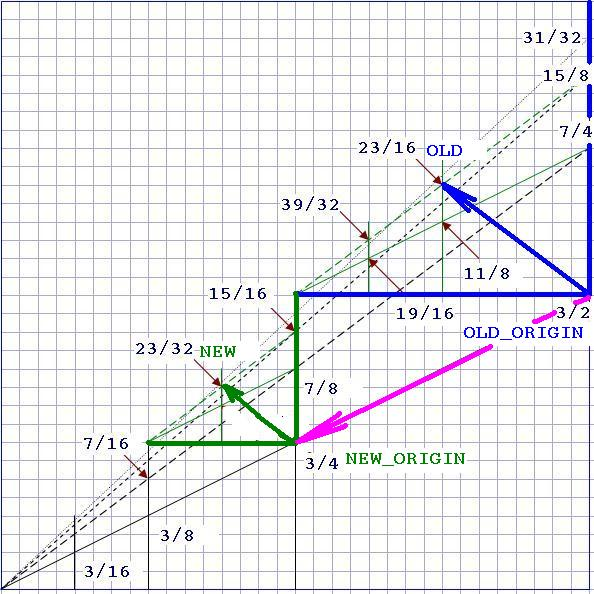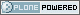# DBAzine.com

##### Personal tools
You are here: Home » Relocating Subtrees in Nested Intervals Model
Seeking new owner for this high-traffic DBAzine.com site.
##### Who Are You?
I am a:
Mainframe True Believer
Distributed Fast-tracker

[ Results | Polls ]

# Relocating Subtrees in Nested Intervals Model

In previous article, I introduced Nested Intervals Model — a tree labeled with Binary Rational Numbers. The advantage of this model is that every node has a permanent label, so insertion of a new node doesn’t affect existing nodes. I’ll describe how to move parts of the hierarchy in the Nested Intervals Model.

## The Problem

Suppose we have a hierarchy:

```select lpad(' ',3*depth)||name, path, numer ||'/index.html'|| denomfrom hierarchy order by numer_left/denom_left
lpad(' ',3*dept path            numer ||'/--------------- --------------- ---------- KING            .1              3/2   CLARK        .1.3            19/16      MILLER    .1.3.1          39/32   BLAKE        .1.2            11/8      TURNER    .1.2.4          163/128      MARTIN    .1.2.3          83/64      WARD      .1.2.2          43/32      ALLEN     .1.2.1          23/16   JONES        .1.1            7/4      FORD      .1.1.2          27/16         SMITH  .1.1.2.1        55/32      SCOTT     .1.1.1          15/8         ADAMS  .1.1.1.1        31/16```

How would we reorganize this tree so that BLAKE doesn’t report to KING anymore, but starts working for MILLER? We require all BLAKE’s descendants in the hierarchy to keep their relative position. In other words, after reorganization, TURNER, MARTIN, WARD, and ALLEN still report to BLAKE.

## The Idea

We'll leverage the fact that every subtree is a scaled up (or down) replica of the other subtree mentioned in the previous article. At the picture below, for example, “green” subtree is identical to the “blue” subtree, reduced twice:More formally, each “green” vector beginning at the NEW_ORIGIN and ending at the NEW point is half of the corresponding “blue” vector that begins at OLD_ORIGIN and ends at the OLD point. This could be written as two equations for X and Y vector components:

`new_x - new_origin_x = k * (old_x - old_origin_x)new_y - new_origin_y = k * (old_y - old_origin_y)`

This is a linear transformation, in which k is a zooming factor (equal to 1/2 in our example). The picture above should convince you that zooming factor k is just the ratio of the origin’s denominators: denominator of the NEW_ORIGIN is equal to 4 while denominator of the OLD_ORIGIN is two.

If we add these equations together, then we have the following:

`(new_x+new_y) - (new_origin_x+new_origin_y) == k * ((old_x+old_y) - (old_origin_x+old_origin_y))`

Now, since those node labeling was defined as the sum of components we simplify it to the following:

`new - new_origin = k * (old - old_origin)`

## Implementation

Once again, we are using integer numbers in our implementation, which is why functions that compute the NEW label are more lengthy than we might expect from such a simple formula. Let’s first introduce two helper functions that would reduce
rational binary number to lower terms:

```function normalize_numer(numer integer, denom integer)
RETURN integer IS
num integer;
den integer;
BEGIN
ret_num := numer;
ret_den := denom;
while floor(ret_num/2) = ret_num/2 loop
ret_num := ret_num/2;
ret_den := ret_den/2;
end loop;
RETURN ret_num;
END;

function normalize__denom(numer integer, denom integer)
...
RETURN ret_den;
END;

select normalize_numer(10,16),normalize_denom(10,16) from dual

5,8
```

Next, the functions that calculate relocated node position:

```function new_numer( old_numer        integer,                      old_denom        integer,                    old_origin_numer integer,                     old_origin_denom integer,                    new_origin_numer integer,                    new_origin_denom integer )RETURN integer IS   ret_num integer;   ret_den integer;   zoom_factor integer;BEGIN   -- special case of a vector in the origin   if  old_numer=old_origin_numer   and old_denom=old_origin_denom then      return new_origin_numer;   end if;   -- case: zoom in or zoom out   if old_origin_denom < new_origin_denom then      zoom_factor := new_origin_denom/old_origin_denom;      -- Subtract old - old_origin first, then multiply      -- Certainly, old_denom > old_origin_denom      ret_num := old_numer - old_origin_numer*                           old_denom /old_origin_denom;      ret_den := old_denom*zoom_factor;   else      zoom_factor := old_origin_denom/new_origin_denom;      ret_num := (old_numer - old_origin_numer*               old_denom/old_origin_denom)*zoom_factor;      ret_den := old_denom;   end if;   ret_num := normalize_numer(ret_num, ret_den);   ret_den := normalize_denom(ret_num, ret_den);     -- apriori we don't know if ret_denom is larger   -- than new_origin_denom or not   if ret_den < new_origin_denom then      ret_num := new_origin_numer +                 ret_num*new_origin_denom/ret_den;      ret_den := new_origin_denom;   else      ret_num := new_origin_numer*                 ret_den/new_origin_denom + ret_num;      ret_den := ret_den;   end if;   ret_num := normalize_numer(ret_num, ret_den);   ret_den := normalize_denom(ret_num, ret_den);     RETURN ret_num;END;
function new_denom( old_numer        integer,                      old_denom        integer,                    old_origin_numer integer,                      old_origin_denom integer,                    new_origin_numer integer,                    new_origin_denom integer )RETURN integer IS    ret_num integer;    ret_den integer;    zoom_factor integer; BEGIN...   return new_origin_denom;...   RETURN ret_den; END; ```

Warm-up testing:

```select new_numer(27,16, 3,2, 3,4)||'/index.html'||new_denom(27,16, 3,2, 3,4) from dual27/32
select new_numer(27,16, 7,4, 3,4)||'/index.html'||new_denom(27,16, 7,4, 3,4) from dual11/16```

## The Final Test

We move all subhierarchy under “BLAKE” into “MILLER” as follows:

```update empsset numer = new_numer(numer,denom,                      11,8,                      child_numer(39,32,1),                      child_denom(39,32,1)),   denom = new_denom(numer,denom,                      11,8,                      child_numer(39,32,1),                      child_denom(39,32,1))where distance(numer,denom, 11,8)>=0
5 rows updated.```

The idea is to find the first child of MILLER, which is labeled as child_numer(39,32,1)/child_denom(39,32,1), and move all the nodes reachable from BLAKE (labeled as 11/8) into the new position.

The resulting hierarchy:

```select lpad(' ',3*depth)||name, path, numer ||'/index.html'|| denomfrom hierarchy order by numer_left/denom_left
lpad(' ',3*dept path            numer ||'/--------------- --------------- ---------- KING            .1              3/2   CLARK        .1.3            19/16      MILLER    .1.3.1          39/32         BLAKE  .1.3.1.1        79 64            TUR .1.3.1.1.4      1251/1024            MAR .1.3.1.1.3      627/512            WAR .1.3.1.1.2      315/256            ALL .1.3.1.1.1      159/128      JONES        .1.1            7/4      FORD      .1.1.2          27/16         SMITH  .1.1.2.1        55/32      SCOTT     .1.1.1          15/8         ADAMS  .1.1.1.1        31/16```

## Acknowledgments

Many thanks to Robin Tucker who posted the problem at comp.databases.theory usenet group and triggered this follow-up article.

--

Vadim Tropashko works for Real World Performance group at Oracle Corp. In prior life he was application programmer and translated "The C++ Programming Language" by B.Stroustrup, 2nd edition into Russian. His current interests include SQL Optimization, Constraint Databases, and Computer Algebra Systems.

Contributors : Vadim Tropashko, Robin Tucker